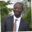Related Tags

js
communitycreator

# How to use forEach() to create a unique values merged array in JSAbel Lifaefi Mbula

### Overview

We use the forEach() method to loop over an array. In this shot, we'll combine the includes() and concat() methods to create a new array with unique values. We'll consider two arrays of strings, from which we want to have an array made of unique values.

Here are the example arrays we'll consider throughout this shot:

const arr1 = ['b', 'c', 'd', 'f', 'g', 'h', 'j', 'k', 'l', 'm', 'n', 'p']
const arr2 = ['b', 'c', 'n', 'p', 'q', 'r', 's', 't', 'v', 'w', 'x', 'z']
The example arrays

Let's look at the function that we'll be using:

const arr1 = ['b', 'c', 'd', 'f', 'g', 'h', 'j', 'k', 'l', 'm', 'n', 'p']
const arr2 = ['b', 'c', 'n', 'p', 'q', 'r', 's', 't', 'v', 'w', 'x', 'z']

function uniqueValuesArr(arr1, arr2) {
const arr3 = []

return arr3
}

// call the function with arrays above and print the output
console.log(uniqueValuesArr(arr1, arr2))
The function to use

The function uniqueValuesArr() that we have just created accepts two parameters that are arrays.

In line 5, we create a new array that we call arr3. This will contain the unique values from the input arrays.

### Loop, push, and concatenate

We use forEach() to loop over an array as you may already know. We first first have to loop over the first array and put the items in the third array if and only if they don't already exist in the second array. Let's just implement it so that you get it.

// inside the function
arr1.forEach(item => {
if(!arr2.includes(item))
arr3.push(item)
})

You can notice in line 3 that I use includes() to check whether a given item in arr1 already exists in arr2. Considering our example, arr3 now becomes:

// inside the funciton
const arr3 = ['d', 'f', 'g', 'h', 'j', 'k', 'l', 'm']

Next, we have to concatenate the third array with the second one.

// inside the funciton
let arr3 = ['d', 'f', 'g', 'h', 'j', 'k', 'l', 'm']

arr3 = arr3.concat(arr2)
• Thanks to the concat() method, we can merge arr3 with arr2.
• Notice also how I changed arr3 variable declaration from const to let. This is because we reassign arr3 (see line 4).

### Run the code

Let's put it all together and run the code to see the output.

const arr1 = ['b', 'c', 'd', 'f', 'g', 'h', 'j', 'k', 'l', 'm', 'n', 'p']
const arr2 = ['b', 'c', 'n', 'p', 'q', 'r', 's', 't', 'v', 'w', 'x', 'z']

function uniqueValuesArr(arr1, arr2) {
let arr3 = []

// loop over arr1 w/ forEach
arr1.forEach(item => {
// push only unique item from arr1 to arr3
if(!arr2.includes(item))
arr3.push(item)
})

arr3 = arr3.concat(arr2)

return arr3.sort()
}

// call the function w/ arrays above and print the output
console.log(uniqueValuesArr(arr1, arr2))
Run the code

Notice how I use the sort() method in line 16 to sort the letters from the merged array alphabetically.

RELATED TAGS

js
communitycreator

CONTRIBUTORAbel Lifaefi Mbula
RELATED COURSES

View all Courses

Keep Exploring

Learn in-demand tech skills in half the time